# Permutation and Combinations (Part - 2) CA CPT Notes | EduRev

## CA CPT : Permutation and Combinations (Part - 2) CA CPT Notes | EduRev

The document Permutation and Combinations (Part - 2) CA CPT Notes | EduRev is a part of the CA CPT Course Business Mathematics and Logical Reasoning & Statistics.
All you need of CA CPT at this link: CA CPT

COMBINATIONS
We have studied about permutations in the earlier section. There we have said that while arranging or selecting, we should pay due regard to order. There are situations in which order is not important. For example, consider selection of 5 clerks from 20 applicants. We will not be concerned about the order in which they are selected. In this situation, how to find the number of ways of selection? The idea of combination applies here.

Definition : The number of ways in which smaller or equal number of things are arranged or selected from a collection of things where the order of selection or arrangement is not important, are called combinations.

The selection of a Poker hand which is a combination of five cards selected from 52 cards is an example of combination of 5 things out of 52 things.

Number of combinations of n different things taken r at a time. (denoted by nCr  C(n,r)  C (n/r ), Cn,r)

Let nCr denote the required number of combinations. Consider any one of those combinations.
It will contain r things. Here we are not paying attention to order of selection. Had we paid attention to this, we will have permutations or r items taken r at a time. In other words, every combination of r things will have rPr permutations amongst them. Therefore, nCr combinations will give rise to nCr. rPr permutations of r things selected form n things. From the earlier section, we can say that nCr. rPr = nPr as nPr denotes the number of permutations of r things chosen out of n things.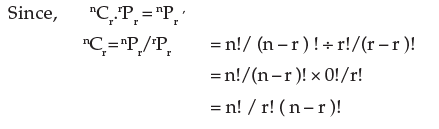Remarks: Using the above formula, we get
(i) nCo = n! / 0! ( n – 0 )! = n!/n! =1. [ As 0! = 1]
nC= n! / n! ( n – n ) ! = n! / n! 0! = 1 [ Applying the formula for nCr  with r = n ]

Example 1 : Find the number of different poker hands in a pack of 52 playing cards.

Solution : This is the number of combinations of 52 cards taken five at a time. Now applying the formula,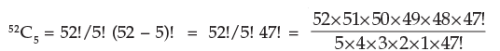= 2,598,960

Example 2 : Let S be the collection of eight points in the plane with no three points on the straight line. Find the number of triangles that have points of S as vertices.

Solution : Every choice of three points out of S determine a unique triangle. The order of the points selected is unimportant as whatever be the order, we will get the same triangle. Hence, the desired number is the number of combinations of eight things taken three at a time.
Therefore, we get
8C3 = 8!/3!5! = 8×7×6/3×2×1 = 56 choices.

Example 3 : A committee is to be formed of 3 persons out of 12. Find the number of ways of forming such a committee.

Solution : We want to find out the number of combinations of 12 things taken 3 at a time and this is given by

12C3 = 12!/3!(12 – 3)! [ by the definition of nCr]
= 12!/3!9! = 12×11×10×9!/3!9! = 12×11×10/3×2 = 220

Example 4 : A committee of 7 members is to be chosen from 6 Chartered Accountants, 4 Economists and 5 Cost Accountants. In how may ways can this be done if in the committee, there must be at least one member from each group and at least 3 Chartered Accountants?

Solution : The various methods of selecting the persons from the various groups are shown below: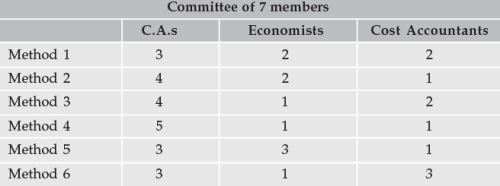Number of ways of choosing the committee members by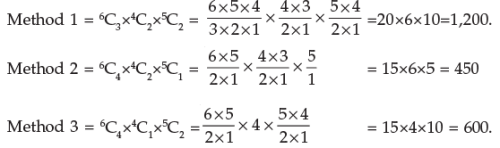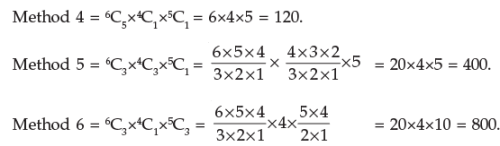Therefore, total number of ways = 1,200 + 450 + 600 + 120 + 400 + 800 = 3,570

Example 5: A person has 12 friends of whom 8 are relatives. In how many ways can he invite 7 guests such that 5 of them are relatives?

Solution : Of the 12 friends, 8 are relatives and the remaining 4 are not relatives. He has to invite 5 relatives and 2 friends as his guests. 5 relatives can be chosen out of 8 in 8C5 ways; 2 friends can be chosen out of 4 in 4C2 ways.

Hence, by the fundamental principle, the number of ways in which he can invite 7 guests such that 5 of them are relatives and 2 are friends.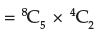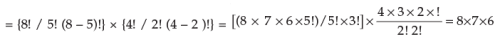= 336.

Example 6 : A Company wishes to simultaneously promote two of its 6 department heads out of 6 to assistant managers. In how many ways these promotions can take place?

Solution : This is a problem of combination. Hence, the promotions can be done in
6C2 = 6×5 / 2 = 15 ways

Example 7 : A building contractor needs three helpers and ten men apply. In how many ways can these selections take place?

Solution : There is no regard for order in this problem. Hence, the contractor can select in any of 10C3 ways i.e.,
(10 × 9 × 8) / (3 × 2 × 1) = 120 ways.

Example 8: In each case, find n:

Solution : (a) 4. nC2 = n+2 C3; (b) n+2 Cn = 45.
(a) We are given that 4. nC2 = n+2 C3. Now applying the formula,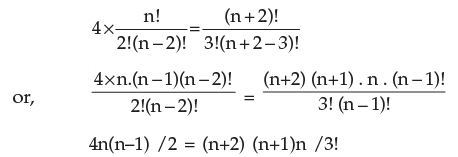or, 4n(n–1) / 2 = (n+2)(n+1)n /3×2×1
or, 12(n–1)=(n+2) (n+1)
or, 12n–12 = n2 + 3n +2
or, n2 – 9n + 14 = 0.
or, n2 – 2n – 7n + 14 = 0.
or, (n–2) (n–7) = 0
∴ n=2   or   7.

(b) We are given that n+2Cn = 45. Applying the formula,
(n+2)!/{n!(n+2–n)!} = 45
or, (n+2) (n+1) n! / n! 2! = 45

or, (n+1) (n+2) = 45×2! = 90
or, n2+3n–88 = 0
or, n2+11n–8n–88 = 0
or, (n+11) (n–8) = 0

Thus, n equals either – 11 or 8. But negative value is not possible. Therefore we conclude that n=8.

Example 9 : A box contains 7 red, 6 white and 4 blue balls. How many selections of three balls can be made so that (a) all three are red, (b) none is red, (c) one is of each colour?

Solution : (a) All three balls will be of red colour if they are taken out of 7 red balls and this can be done in
7C3 = 7! / 3!(7–3)!
= 7! / 3!4! = 7×6×5×4! / (3×2×4!) = 7×6×5 / (3×2) = 35 ways
Hence, 35 selections (groups) will be there such that all three balls are red.
(b) None of the three will be red if these are chosen from (6 white and 4 blue balls) 10 balls and this can be done in
10C3 =  10!/{3!(10–3)!} = 10! / 3!7!
=  10×9×8×7! / (3×2×1×7!) = 10×9×8 /(3×2) = 120 ways.
Hence, the selections (or groups) of three such that none is red ball are 120 in number.
One red ball can be chosen from 7 balls in 7C1 = 7 ways. One white ball can be chosen from 6 white balls in 6C1 ways. One blue ball can be chosen from 4 blue balls in 4C= 4 ways. Hence, by generalized fundamental principle, the number of groups of three balls such that one is of each colour = 7×6×4 = 168 ways.

Example 10 : If 10Pr = 604800 and 10Cr = 120; find the value of r,

Solution : We know that nCr. rPr = nPr. We will us this equality to find r.
10Pr = 10C.r!
or, 604800 = 120 ×r!
or, r! = 604800 ÷ 120 = 5040
But r! = 5040 = 7×6×4×3×2×1 = 7!
Therefore, r=7.

Properties of nCr :
1. nCr = nCn–r
We have nC= n! / {r!(n–r)!} and nCn–r = n! / [(n–r)! {n–(n–r)}!] = n! / {(n–r)!(n–n+r)!}
Thus nCn–r = n! / {(n–r)! (n–n+r)!} = n! / {(n–r)!r!} = nCr

2. n+1C= nC+ nCr–1

By definition,
nCr–1 + nCr = n! / {(r–1)! (n–r+1)!} + n! / {r!(n–r)!}
But r! = r×(r–1)! and (n–r+1)! = (n–r+1) × (n–r)!. Substituting these in above, we get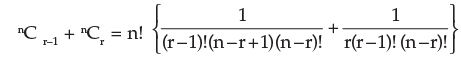= {n! / (r–1)! (n–r)!} {(1 / n–r+1) + (1/r) }
= {n! / (r–1)! (n–r)!} {(r+n–r+1) / r(n–r+1) }
= (n+1) n! / {r . (r–1)! (n–r)! . (n–r+1)}
= (n+1)! / {r!(n+1–r)!} = n+1Cr

3. nCo = n!/{0! (n–0)!} = n! / n! =1.
4. nCn = n!/{n! (n–n)!} = n! / n! . 0! = 1.

Note
(a) nCr has a meaning only when 0≤ r ≤ n, nCn–r has a meaning only when 0 ≤ n – r ≤ n.
(b) nCr and nCn–r are called complementary combinations, for if we form a group of r things out of n different things, (n–r) remaining things which are not included in this group form another group of rejected things. The number of groups of n different things, taken r at a time should be equal to the number of groups of n different things taken (n–r) at a time.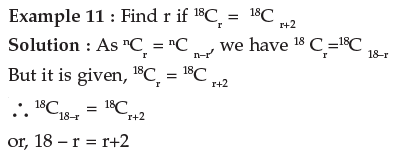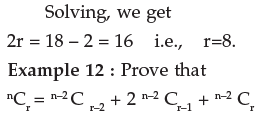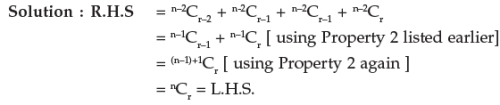Hence, the result

Example 13 : If 28C2r : 24C2r–4 = 225 : 11, find r.

Solution : We have nCr = n! / {r!(n–r)!} Now, substituting for n and r, we get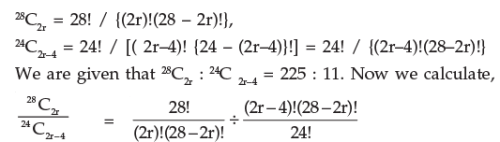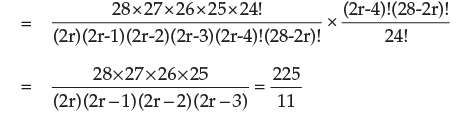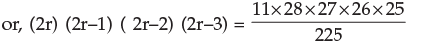= 11×28×3×26
= 11×7×4×3×13×2
= 11×12×13×14
= 14×13×12×11
∴   2r= 14 i.e., r = 7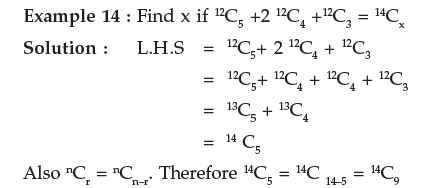Hence, L.H.S = 14C5 = 14C= 14Cx = R.H.S by the given equality
This implies, either x = 5 or x = 9.

Example 15 : Prove by reasoning that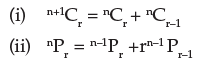Solution : (i) n+1Cr stands for the number of combinations of (n+1) things taken r at a time. As a specified thing can either be included in any combination or excluded from it, the total number of combinations which can be combinations or (n+1) things taken r at a time is the sum of :

(a) combinations of (n+1) things taken r at time in which one specified thing is always included and
(b) the number of combinations of (n+1) things taken r at time from which the specified thing is always excluded.
Now, in case (a), when a specified thing is always included , we have to find the number of ways of selecting the remaining (r–1) things out of the remaining n things which is nCr–1.
Again, in case (b), since that specified thing is always excluded, we have to find the number of ways of selecting r things out of the remaining n things,  which is nCr.
Thus, n+1Cr = nCr–1+ nCr
(i) We devide nPi.e., the number of permutations of n things take r at a time into two groups:
(a) those which contain a specified thing
(b) those which do not contain a specified thing.
In (a) we fix the particular thing in any one of the r places which can be done in r ways and then fill up the remaining (r–1) places out of (n–1) things which give rise to n–1 Pr–1 ways. Thus, the number of permutations in case (a) = r × n–1Pr–1.
In case (b), one thing is to be excluded; therefore, r places are to be filled out of (n–1) things.
Therefore, number of permutations = n–1Pr
Thus, total number of permutations = n–1Pr + r. n–1Pr–1
i.e.,   nPr = n–1Pr+r. n–1Pr–1

STANDARD RESULTS

We now proceed to examine some standard results in permutations and combinations. These results have special application and hence are dealt with separately.

I. Permutations when some of the things are alike, taken all at a time
The number of ways p in which n things may be arranged among themselves, taking them all at a time, when n1 of the things are exactly alike of one kind , nof the things are exactly alike of another kind, n3 of the things are exactly alike of the third kind, and the rest all are different is given by,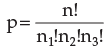Proof : Let there be n things. Suppose n1 of them are exactly alike of one kind; n2 of them are exactly alike of another kind; n3 of them are exactly alike of a third kind; let the rest (n–n1–n2–n3) be all different.
Let p be the required permutations; then if the n things, all exactly alike of one kind were replaced by n, different things different from any of the rest in any of the p permutations without altering the position of any of the remaining things, we could form n1! new permutations.
Hence, we should obtain p × n1! permutations.
Similarly if n2 things exactly alike of another kind were replaced by n2 different things different form any of the rest, the number of permutations would be p × n1! × n2!

Similarly, if n3 things exactly alike of a third kind were replaced by n3 different things different from any of the rest, the number of permutations would be p × n1! × n2! × n3! = n!
But now because of these changes all the n things are different and therefore, the possible number of permutations when all of them are taken is n!.
Hence,   p×n1! × n2! n3! = n!

i.e.,which is the required number of permutations. This results may be extended to cases where there are different number of groups of alike things.

II. Permutations when each thing may be repeated once, twice,…upto r times in any arrangement.

Result: The number of permutations of n things taken r at time when each thing may be repeated r times in any arrangement is nr.

Proof: There are n different things and any of these may be chosen as the first thing. Hence, there are n ways of choosing the first thing.
When this is done, we are again left with n different things and any of these may be chosen as the second (as the same thing can be chosen again.) Hence, by the fundamental principle, the two things can be chosen in n × n = nnumber of ways.
Proceeding in this manner, and noting that at each stage we are to chose a thing from n different things, the total number of ways in which r things can be chosen is obviously equal to n × n × ………to r terms = nr.

III. Combinations of n different things taking some or all of n things at a time

Result : The total number of ways in which it is possible to form groups by taking some or all of n things (2n –1).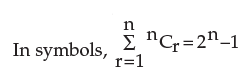Proof : Each of the n different things may be dealt with in two ways; it may either be taken or left. Hence, by the generalised fundamental principle, the total number of ways of dealing with n things :
2 × 2 × 2×……..2, n times i.e., 2n
But this include the case in which all the things are left, and therefore, rejecting this case, the total number of ways of forming a group by taking some or all of n different things is 2n – 1.
IV. Combinations of n things taken some or all at a time when n1 of the things are alike of one kind, n2 of the things are alike of another kind n3 of the things are alike of a third kind. etc.

Result : The total, number of ways in which it is possible to make groups by taking some or all out of n (=n1 + n2 + n3 +…) things, where n1 things are alike of one kind and so on, is given by {(n1 + 1) ( n+ 1) ( n+ 1)…} –1
Proof : The n1 things all alike of one kind may be dealt with in (n+ 1) ways. We may take 0, 1, 2,….n, of them. Similarly n2 things all alike of a second kind may be dealt with in (n2 +1) ways and n3 things all alike of a third kind may de dealt with in (n3 +1) ways.
Proceeding in this way and using the generalised fundamental principle, the total number of ways of dealing with all n ( = n1 + n2 + n3 +…) things, where n1, things are alike of one kind and so on, is given by (n1 + 1) ( n2 + 1) ( n+ 1)…
But this includes the case in which none of the things are taken. Hence, rejecting this case, total number of ways is {(n1 + 1) ( n2 + 1) ( n3 + 1)…} –1}

V. The notion of Independence in Combinations

Many applications of Combinations involve the selection of subsets from two or more independent sets of objects or things. If the combination of a subset having r1 objects form a set having n1 objects does not affect the combination of a subset having r2 objects from a different set having nobjects, we call the combinations as being independent. Whenever such combinations are independent, any subset of the first set of objects can be combined with each subset of the second set of the object to form a bigger combination. The total number of such combinations can be found by applying the generalised fundamental principle.
Result : The combinations of selecting r1 things from a set having nobjects and rthings from a set having n2 objects where combination of rthings, r2 things are independent is given by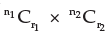Note : This result can be extended to more than two sets of objects by a similar reasoning.

Example 1 : How many different permutations are possible from the letters of the word CALCULUS?

Solution: The word CALCULUS consists of 8 letters of which 2 are C and 2 are L, 2 are U and the rest are A and S. Hence , by result (I), the number of different permutations from the letters of the word CALCULUS taken all at a time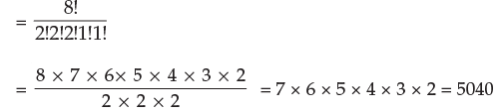Example 2 : In how many ways can 17 billiard balls be arranged , if 7 of them are black, 6 red and 4 white?

Solution : We have, the required number of different arrangements: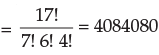Example 3 : An examination paper with 10 questions consists of 6 questions in Algebra and 4 questions in Geometry. At least one question from each section is to be attempted. In how many ways can this be done?

Solution : A student must answer atleast one question from each section and he may answer all questions from each section.
Consider Section I : Algebra. There are 6 questions and he may answer a question or may not answer it. These are the two alternatives associated with each of the six questions. Hence, by the generalised fundaments principle, he can deal with two questions in 2 × 2 ….6 factors = 26 number of ways. But this includes the possibility of none of the question from Algebra being attempted. This cannot be so, as he must attempt at least one question from this section. Hence, excluding this case, the number of ways in which Section I can be dealt with is (26 –1).
Similarly, the number of ways in which Section II can be dealt with is (24 –1).

Hence, by the Fundamental Principle, the examination paper can be attempted in (26 –1) (24 –1) number of ways.

Example 4 : A man has 5 friends. In how many ways can he invite one or more of his friends to dinner?

Solution : By result, (III) of this section, as he has to select one or more of his 5 friends, he can do so in 25 – 1 = 31 ways.
Note : This can also be done in the way, outlines below. He can invite his friends one by one, in twos, in threes, etc. and hence the number of ways.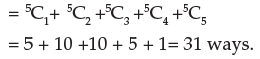Example 5 : There are 7 men and 3 ladies. Find the number of ways in which a committee of 6 can be formed of them if the committee is to include atleast two ladies?

Solution : The committee of six must include at least 2 ladies, i.e., two or more ladies. As there are only 3 ladies, the following possibilities arise: The committee of 6 consists of (i) 4 men and 2 ladies (ii) 3 men and 3 ladies.
The number of ways for (i) = 7C4 × 3C2 = 35 × 3 = 105;
The number of ways for (ii) = 7C3 × 3C3 = 35 × 1 = 35.
Hence the total number of ways of forming a committee so as to include at least two ladies = 105 +35 = 140.

Example 6 : Find the number of ways of selecting 4 letters from the word EXAMINATION.

Solution : There are 11 letters in the word of which A, I, N are repeated twice.

Thus we have 11 letters of 8 different kinds (A, A), (I, I), (N, N), E, X, M, T, O.
The group of four selected letters may take any of the following forms:
(i) Two alike and other two alike
(ii) Two alike and other two different
(iii) All four different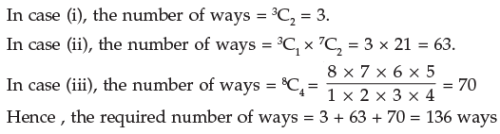Offer running on EduRev: Apply code STAYHOME200 to get INR 200 off on our premium plan EduRev Infinity!

69 docs|76 tests

,

,

,

,

,

,

,

,

,

,

,

,

,

,

,

,

,

,

,

,

,

;